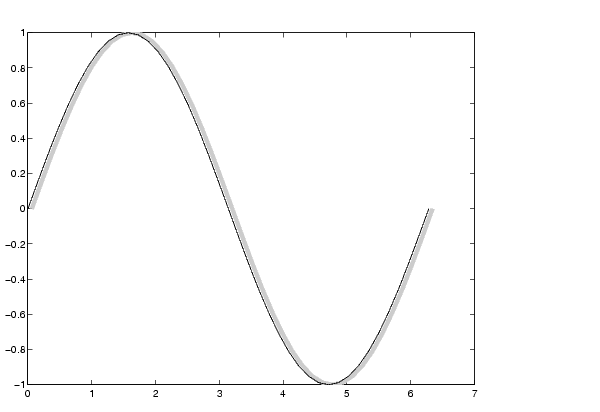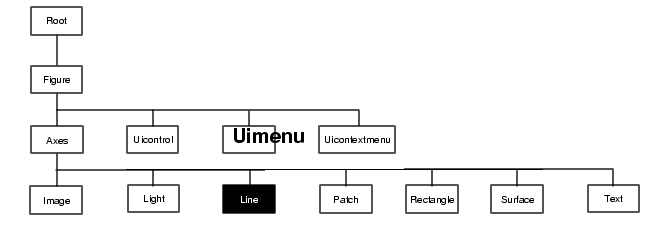MATLAB Function Referenceline

Create line object

Syntax

• ```line(X,Y)
line(X,Y,Z)
line(X,Y,Z,'PropertyName',PropertyValue,...)
line('PropertyName',PropertyValue,...) low-level-PN/PV pairs only
h = line(...)
```

Description

`line` creates a line object in the current axes. You can specify the color, width, line style, and marker type, as well as other characteristics.

The `line` function has two forms:

• Automatic color and line style cycling. When you specify matrix coordinate data using the informal syntax (i.e., the first three arguments are interpreted as the coordinates),
• ``````line(X,Y,Z)
``````
• MATLAB cycles through the axes `ColorOrder` and `LineStyleOrder` property values the way the `plot` function does. However, unlike `plot`, `line` does not call the `newplot` function.

• Purely low-level behavior. When you call `line` with only property name/property value pairs,
• ``````line('XData',x,'YData',y,'ZData',z)
``````
• MATLAB draws a line object in the current axes using the default line color (see the `colordef` function for information on color defaults). Note that you cannot specify matrix coordinate data with the low-level form of the `line` function.

```line(X,Y) ``` adds the line defined in vectors `X` and `Y` to the current axes. If `X` and `Y` are matrices of the same size, `line` draws one line per column.

```line(X,Y,Z) ``` creates lines in three-dimensional coordinates.

```line(X,Y,Z,'PropertyName',PropertyValue,...) ``` creates a line using the values for the property name/property value pairs specified and default values for all other properties.

See the `LineStyle` and `Marker` properties for a list of supported values.

```line('XData',x,'YData',y,'ZData',z,'PropertyName',PropertyValue,.. .) ``` creates a line in the current axes using the property values defined as arguments. This is the low-level form of the `line` function, which does not accept matrix coordinate data as the other informal forms described above.

```h = line(...) ``` returns a column vector of handles corresponding to each line object the function creates.

Remarks

In its informal form, the `line` function interprets the first three arguments (two for 2-D) as the `X`, `Y`, and `Z` coordinate data, allowing you to omit the property names. You must specify all other properties as name/value pairs. For example,

• ```line(X,Y,Z,'Color','r','LineWidth',4)
```

The low-level form of the `line` function can have arguments that are only property name/property value paris. For example,

• ````line`('XData',x,'YData',y,'ZData',z,'Color','r','LineWidth',4)
```

Line properties control various aspects of the line object and are described in the "Line Properties" section. You can also set and query property values after creating the line using `set` and `get`.

You can specify properties as property name/property value pairs, structure arrays, and cell arrays (see the `set` and `get` reference pages for examples of how to specify these data types).

Unlike high-level functions such as `plot`, `line` does not respect the setting of the figure and axes `NextPlot` properties. It simply adds line objects to the current axes. However, axes properties that are under automatic control such as the axis limits can change to accommodate the line within the current axes.

Examples

This example uses the `line` function to add a shadow to plotted data. First, plot some data and save the line's handle:

• ```t = 0:pi/20:2*pi;
hline1 = plot(t,sin(t),'k');
```

Next, add a shadow by offsetting the x coordinates. Make the shadow line light gray and wider than the default `LineWidth`:

• ```hline2 = line(t+.06,sin(t),'LineWidth',4,'Color',[.8 .8 .8]);
```

Finally, pop the first line to the front:

• ```set(gca,'Children',[hline1 hline2])```

Input Argument Dimensions - Informal Form

This statement reuses the one column matrix specified for `ZData` to produce two lines, each having four points.

• ```line(rand(4,2),rand(4,2),rand(4,1))
```

If all the data has the same number of columns and one row each, MATLAB transposes the matrices to produce data for plotting. For example,

• ```line(rand(1,4),rand(1,4),rand(1,4))
```

is changed to:

• ```line(rand(4,1),rand(4,1),rand(4,1))
```

This also applies to the case when just one or two matrices have one row. For example, the statement,

• ```line(rand(2,4),rand(2,4),rand(1,4))
```

is equivalent to:

• ```line(rand(4,2),rand(4,2),rand(4,1))
```

`axes`,`newplot`, `plot`, `plot3`

Object Creation Functions for related functions

Object HierarchySetting Default Properties

You can set default line properties on the axes, figure, and root levels.

• ```set(0,'DefaultLinePropertyName',PropertyValue,...)
set(gcf,'DefaultLinePropertyName'`,`PropertyValue,...)
set(gca,'DefaultLinePropertyName',PropertyValue,...)
```

Where `PropertyName` is the name of the line property and `PropertyValue` is the value you are specifying. Use `set` and `get` to access line properties.

The following table lists all light properties and provides a brief description of each. The property name links take you to an expanded description of the properties.

 Property Name Property Description Property Value Data Defining the Object `XData` The x-coordinates defining the line Values: vector or matrixDefault: `[0 1]` `YData` The y-coordinates defining the line Values: vector or matrixDefault: `[0 1]` ZData The z-coordinates defining the line Values: vector or matrixDefault: `[]` empty matrix Defining Line Styles and Markers `LineStyle` Select from five line styles. Values: -, --, `:`, -`.`,` none`Default: - `LineWidth` The width of the line in points Values: scalarDefault: `0.5` points `Marker` Marker symbol to plot at data points Values: see `Marker` propertyDefault: `none` `MarkerEdgeColor` Color of marker or the edge color for filled markers Values: `ColorSpec`, `none`, `auto`Default: `auto` `MarkerFaceColor` Fill color for markers that are closed shapes Values: `ColorSpec`, `none`, `auto`Default: `none` `MarkerSize` Size of marker in points Values: size in pointsDefault: `6` Controlling the Appearance Clipping Clipping to axes rectangle Values: `on`, `off`Default: `on` EraseMode Method of drawing and erasing the line (useful for animation) Values: `normal`, `none`, `xor`, `background`Default: `normal` `SelectionHighlight` Highlight line when selected (`Selected` property set to `on`) Values: `on`, `off` Default: `on` `Visible` Make the line visible or invisible Values: `on`, `off` Default: `on` Color Color of the line `ColorSpec` Controlling Access to Objects `HandleVisibility` Determines if and when the the line's handle is visible to other functions Values: `on`, `callback`, `off`Default: `on` `HitTest` Determines if the line can become the current object (see the figure `CurrentObject` property) Values: `on`, `off` Default: `on` General Information About the Line `Children` Line objects have no children Values: `[]` (empty matrix) `Parent` The parent of a line object is always an axes object Value: axes handle `Selected` Indicate whether the line is in a "selected" state. Values: `on`, `off` Default: `on` `Tag` User-specified label Value: any string Default:` '' `(empty string) `Type` The type of graphics object (read only) Value: the string `'line'` `UserData` User-specified data Values: any matrixDefault:` [] `(empty matrix) Properties Related to Callback Routine Execution `BusyAction` Specify how to handle callback routine interruption Values: `cancel`, `queue`Default: `queue` `ButtonDownFcn` Define a callback routine that executes when a mouse button is pressed on over the line Values: string or function handleDefault: `'' `(empty string) `CreateFcn` Define a callback routine that executes when a line is created Values: string or function handleDefault: `'' `(empty string) `DeleteFcn` Define a callback routine that executes when the line is deleted (via `close` or `delete`) Values: string or function handleDefault: `'' `(empty string) `Interruptible` Determine if callback routine can be interrupted Values: `on`, `off` Default: `on` (can be interrupted) `UIContextMenu` Associate a context menu with the line Values: handle of a Uicontextmenulin2mu Line Properties